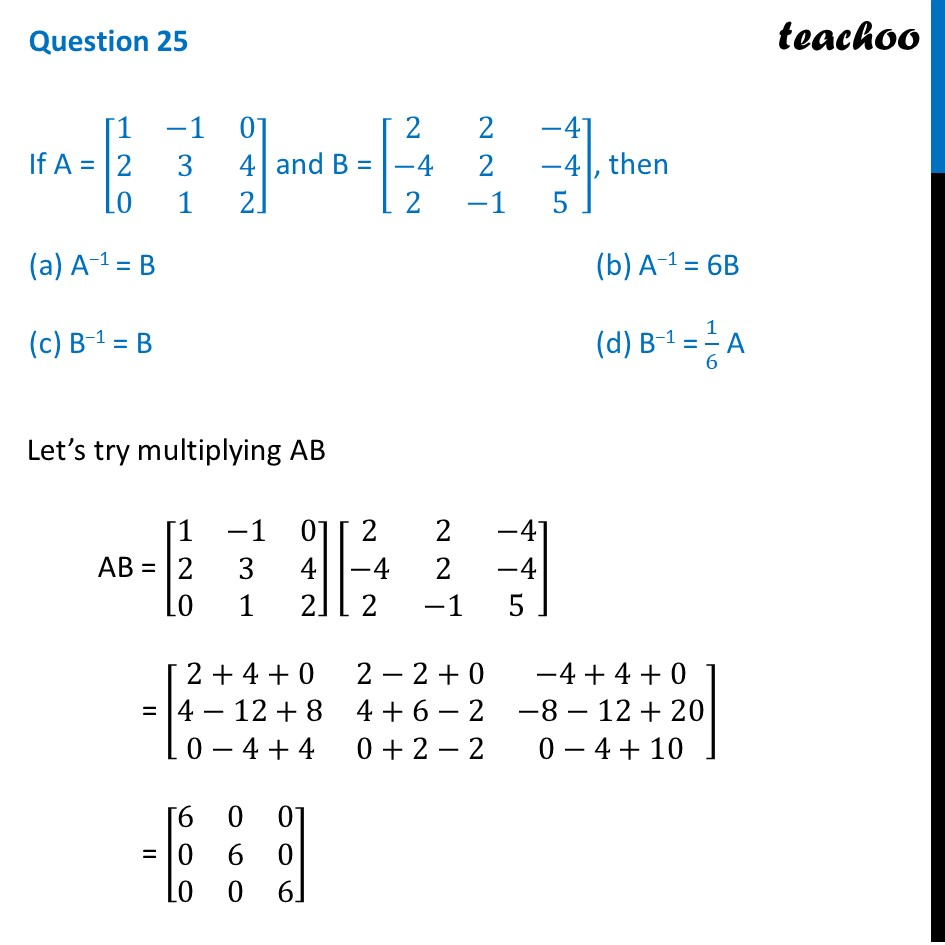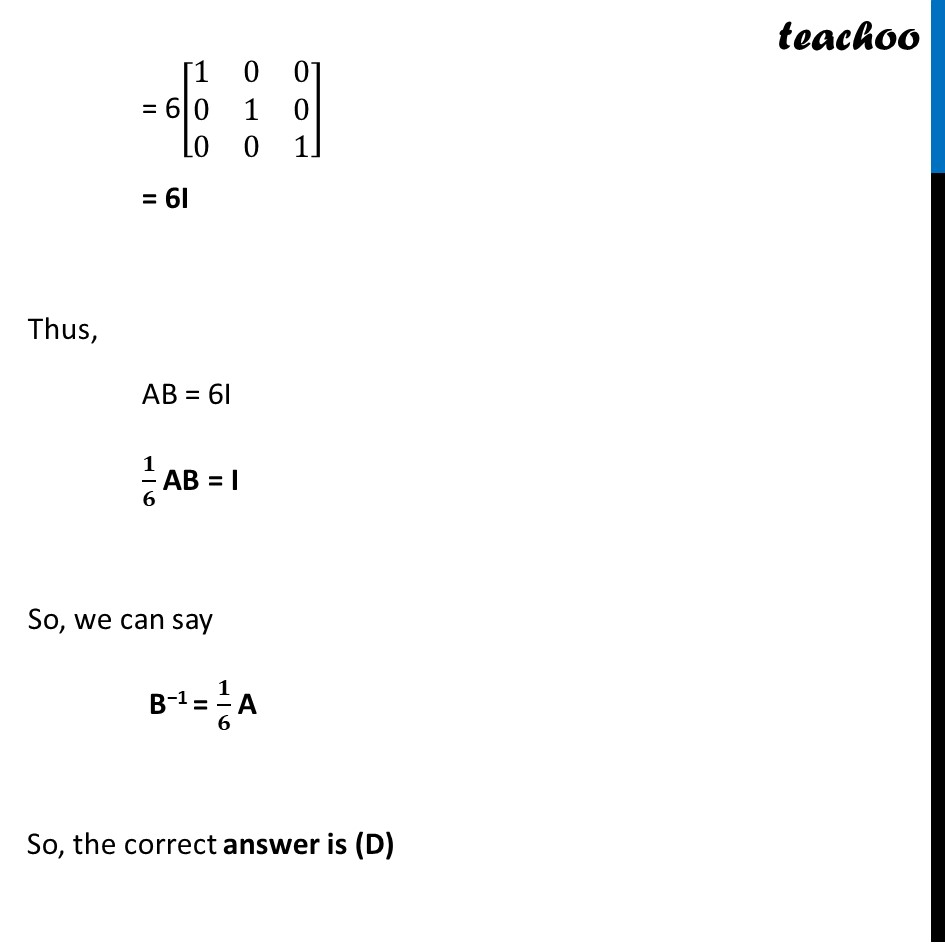CBSE Class 12 Sample Paper for 2022 Boards (MCQ Based - for Term 1)

Class 12
Solutions of Sample Papers and Past Year Papers - for Class 12 Boards

## If A = [8(1 -1 0 2 3 4 0 1 2)] and B = [8(2 2 -4 -4 2 -4 2 -1 5)], then (a) A−1 = B          (b) A−1 = 6B  (c) B−1 = B          (d) B−1 = 1/6 AGet live Maths 1-on-1 Classs - Class 6 to 12

### Transcript

Question 25 If A = [■8(1&−1&[email protected]&3&[email protected]&1&2)] and B = [■8(2&2&−[email protected]−4&2&−[email protected]&−1&5)], then (a) A−1 = B (b) A−1 = 6B (c) B−1 = B (d) B−1 = 1/6 A Let’s try multiplying AB AB = [■8(1&−1&[email protected]&3&[email protected]&1&2)] [■8(2&2&−[email protected]−4&2&−[email protected]&−1&5)] = [■8(2+4+0&2−2+0&−[email protected]−12+8&4+6−2&−8−[email protected]−4+4&0+2−2&0−4+10)] = [■8(6&0&[email protected]&6&[email protected]&0&6)] = 6[■8(1&0&[email protected]&1&[email protected]&0&1)] = 6I Thus, AB = 6I 𝟏/𝟔 AB = I So, we can say B−1 = 𝟏/𝟔 A So, the correct answer is (D)